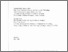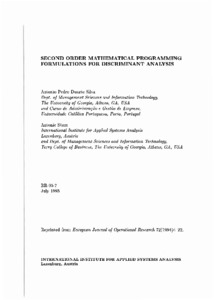Second Order Mathematical Formulations Programming for Discriminant Analysis

Duarte Silva, A.P. & Stam, A. (1994). Second Order Mathematical Formulations Programming for Discriminant Analysis. IIASA Research Report (Reprint). IIASA, Laxenburg, Austria: RR-95-007. Reprinted from European Journal of Operational Research, 72:4-22 .Preview
Text
RR-95-07.pdf - Published Version

Abstract

This paper introduces a nonparametric formulation-based mathematical programming (MP) for solving the classification problem in discriminant analysis. This method differs from previously proposed MP-based models in that, even though the final discriminant function is linear in terms of the parameters to be estimated, the formulation is quadratic in terms of the predictor (attribute) variables. By including second order (i.e., quadratic and cross-product) terms of the attribute variables, the model is similar in concept to the usual treatment of multiple predictor variables in statistical methods such as Fisher's linear discriminant analysis, and allows an analysis of how including nonlinear terms and interaction affect the predictive ability of the estimated classification function. Using simulation experiments involving data conditions for which nonlinear classifiers are appropriate, the classificatory performance of this class of second order MP models is compared with that of existing statistical (linear and quadratic) and first order MP-based formulations. The results of these experiments show that the proposed formulation appears to be a very attractive alternative to previously introduced linear and quadratic statistical and linear MP-based classification methods.

Item Type: Monograph (IIASA Research Report (Reprint)) Methodology of Decision Analysis (MDA) Reprinted from European Journal of Operational Research; 72:4-22  IIASA Import 15 Jan 2016 02:03 27 Aug 2021 17:14 http://pure.iiasa.ac.at/4068View Item